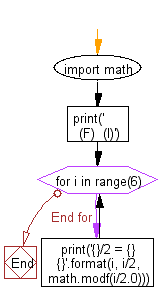﻿ Python Math: Split fractional and integer parts of a floating point number - w3resource# Python Math: Split fractional and integer parts of a floating point number

## Python Math: Exercise-59 with Solution

Write a Python program to split fractional and integer parts of a floating point number.

Sample Solution:-

Python Code:

``````import math
print('           (F)  (I)')
for i in range(6):
print('{}/2 = {} {}'.format(i, i/2, math.modf(i/2.0)))
```
```

Sample Output:

```           (F)  (I)
0/2 = 0.0 (0.0, 0.0)
1/2 = 0.5 (0.5, 0.0)
2/2 = 1.0 (0.0, 1.0)
3/2 = 1.5 (0.5, 1.0)
4/2 = 2.0 (0.0, 2.0)
5/2 = 2.5 (0.5, 2.0)
```

Flowchart:## Visualize Python code execution:

The following tool visualize what the computer is doing step-by-step as it executes the said program:

Python Code Editor:

Have another way to solve this solution? Contribute your code (and comments) through Disqus.

What is the difficulty level of this exercise?

Test your Programming skills with w3resource's quiz.

﻿

```>>> students = [{'name': 'John', 'score': 98}, {'name': 'Mike', 'score': 94}, {'name': 'Jennifer', 'score': 99}]